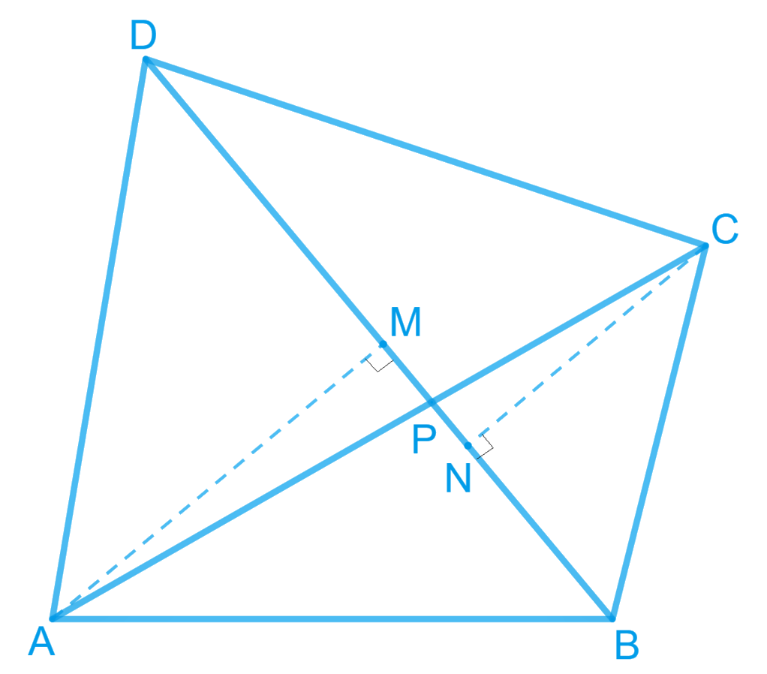# Ex.9.4 Q6 Areas of Parallelograms and Triangles Solution - NCERT Maths Class 9

Go back to  'Ex.9.4'

## Question

Diagonals $$AC$$ and $$BD$$ of a quadrilateral $$ABCD$$ intersect each other at $$P$$. Show that

\begin{align}&{\rm{ ar }}\left( {\Delta APB} \right) \times {\rm{ ar }}\left( {\Delta CPD} \right) \\ &= {\rm{ ar }}\left( {\Delta APD} \right) \times {\rm{ ar }}\left( {\Delta BPC}\right)\end{align}

[Hint: From $$A$$ and $$C$$, draw perpendiculars to $$BD$$]

Video Solution
Areas Of Parallelograms And Triangles
Ex 9.4 | Question 6

## Text Solution

What is known?

A quadrilateral ABCD, in which diagonals AC and BD intersect each other at point P.

What is unknown?

To Prove:

\begin{align}&{\rm{ ar }}\left( {\Delta APB} \right) \times {\rm{ ar }}\left( {\Delta CPD} \right) \\ &= {\rm{ ar }}\left( {\Delta APD} \right) \times {\rm{ ar }}\left( {\Delta BPC}\right)\end{align}

Reasoning:

From A, draw $$AM\bot BD$$ and from C, draw $$CN\bot BD.$$ Now we can find area of triangles to get the required result.Steps:

Proof :

\begin{align} \text{ ar} (\Delta ABP) & \!\!= \!\frac{1}{2} \!\!\times PB \!\times \! AM...\text{(i)} \\ \text{ar} (\Delta APD) & \!\!=\!\frac{1}{2} \!\times \! PD \! \times \! AM...\text{(ii)} \end{align}

Dividing eq. (ii) by (i), we get,

\begin{align} \frac{\text{ar} (\Delta \text{APD)}}{\text{ar} (\Delta ABP)} & =\frac{\frac{1}{2} \times PD\times AM}{\frac{1}{2}\times PB\times AM} \\ \Rightarrow \frac{\text{ar}(\Delta APD)}{\text{ar}(\Delta ABP)}&=\frac{PD}{PB}.....(\text{iii}) \\ \end{align}

Similarly,

\begin{align} & \frac{\text{ar}(\Delta CDP)}{\text{ar}(\Delta BPC)}=\frac{PD}{PB}....(\text{iv}) \end{align}

From eq . (iii) and (iv), we get

\begin{align} & \frac{\text{ar}(\Delta APD)}{\text{ar}(\Delta ABP)}=\frac{\text{ar}(\Delta CDP)}{\text{ar} (\Delta BPC)} \\ & \Rightarrow \text{ar} (\Delta APD)\times \text{ar}(\Delta BPC) \\ & \quad =\text{ar}(\Delta ABP) \times \text{ar} (\Delta CDP) \end{align}

Hence proved.

Video Solution
Areas Of Parallelograms And Triangles
Ex 9.4 | Question 6

Learn from the best math teachers and top your exams

• Live one on one classroom and doubt clearing
• Practice worksheets in and after class for conceptual clarity
• Personalized curriculum to keep up with school